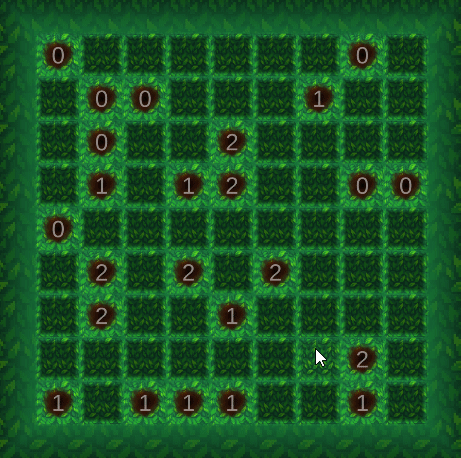# FIBONACCI PRIME COMPOSITE USING C++!!!

|PRIME NUMBER||COMPOSITE NUMBER||FIBONACCI SERIES|

In this program, we going to check whether the given value is a Prime or Composite number and the Fibonacci series.

• Let’s start the program with the header file

“#include<iostream>” →input-output continuous bytes of data.

“#include<stdlib.h>” → stands for Standard Library. It has the information of memory allocation/freeing functions.

“using namespace std;” →used to store variable value.

“void primechk(int a)”

*primechk →function name.

*void →returns data type.

*int a →argument of a (here a is variable);formal parameter.

• Next, we have declared j in integer; j is the local variable because we have defined that inside the primechk so, we can use j only inside the function.

## Prime and Composite Numbers:

A prime number is a number which has exactly two factors i.e. '1' and number itself. A composite number has more than two factors, which means apart from getting divided by number 1 and itself, it can also be divided by at least one integer or number.

• At 1st we are using “if condition”; in if condition we have given a is equal to “0” (||)a is equal to “1”

|| → or operator.

• If we enter 0 (or) 1 the output will be displayed as neither prime nor composite.

“else { for(j=2;j<a;j++)

{ if(a%j==0)

{ cout<<”\t COMPOSITE”;

break;} }

if(a==j)

cout<<”\t PRIME”; } }”

• Now else part, except 0 or 1 if we give any other number it will go inside the else part.

## LOGIC OF THE CODE:

→so, it will again go into for loop.

Upto this the program is about prime and composite.

• Now we get into Fibonacci series.

FIBONACCI SERIES:

The Fibonacci sequence is a series of numbers where a number is the addition of the last two numbers, starting with 0, and 1. The Fibonacci Sequence: 0, 1, 1, 2, 3, 5, 8, 13, 21, 34, 55

“void fibo(int n)”

fibo →function name.

“{ int a=-1,b=1,c=0;

for(int i=1;i<=n;i++)

{ cout<<endl;

c=a+b;

cout<<c;

primechk(c);

a=b;

b=c; } }”

## LOGIC OF THE CODE:

• Now get c=0 in the logic we have to check whether this 0 is prime or not ; c is the fibonacci result.
• Next, we going to control both prime, composite and fibonacci in main program.

“int main()

{ int n;

cout<<”ENTER THE NUMBER OF REQUIRED FIBO TERMS”;

cin>>n;

cout<<”\n\tFIBONACCI SERIES\n”;

fibo(n);

return 0; }”

• Given print staements are , “ENTER THE NUMBER OF REQUIRED FIBO TERMS and FIBONACCI SERIES”.

“fibo(n)” →calling fibonacci series function.

--

--

--

## More from Gomathi Keerthana.R.S.

Love podcasts or audiobooks? Learn on the go with our new app.

## GoTezos Q4 Update## HOW WE BUILD THE GOOGLE DIGITAL GARAGE CLONE?## Teamwork makes the dream work## Creating MineField In Unity (Part 1)## Let’s examine the pros and cons of the Singleton design pattern## Windows 10 Multiple Desktops## Find your purpose in Web3 through Vincent Van Gogh’s life## Pleased to celebrate our new relationship with Eurovia UK to support their digital infrastructure…## Pattern Matching (Regex)## Saving RTSP Camera Streams with FFmpeg